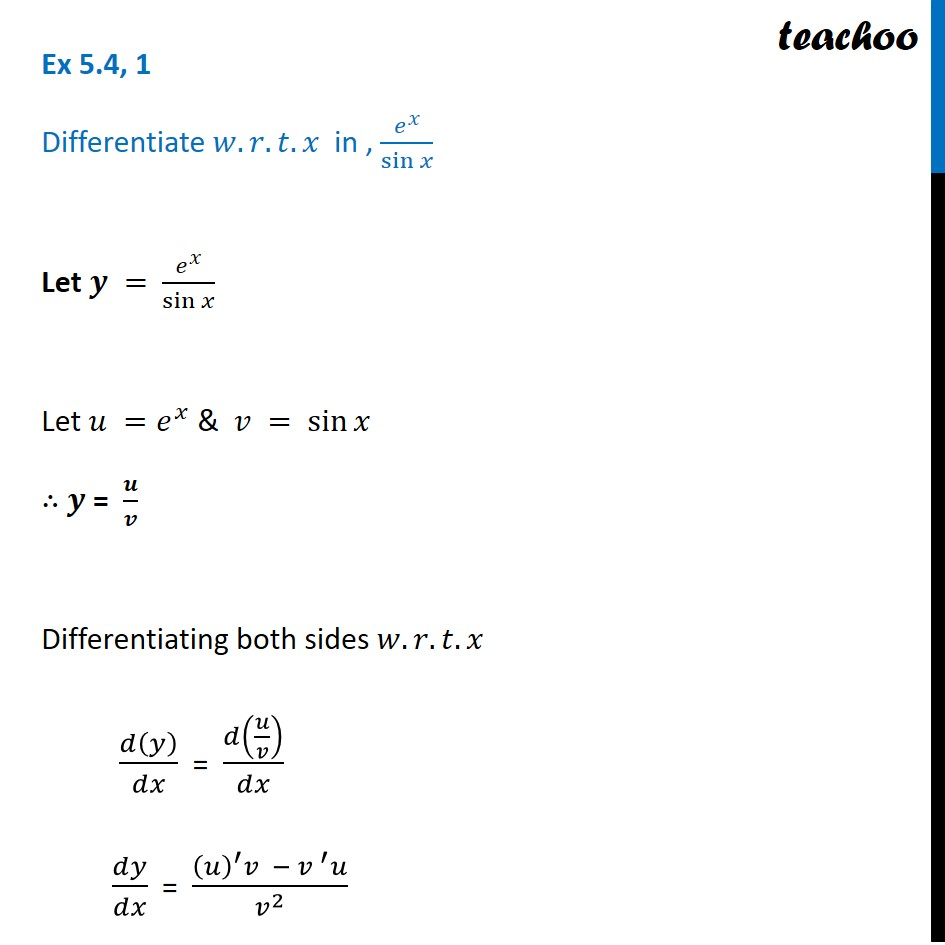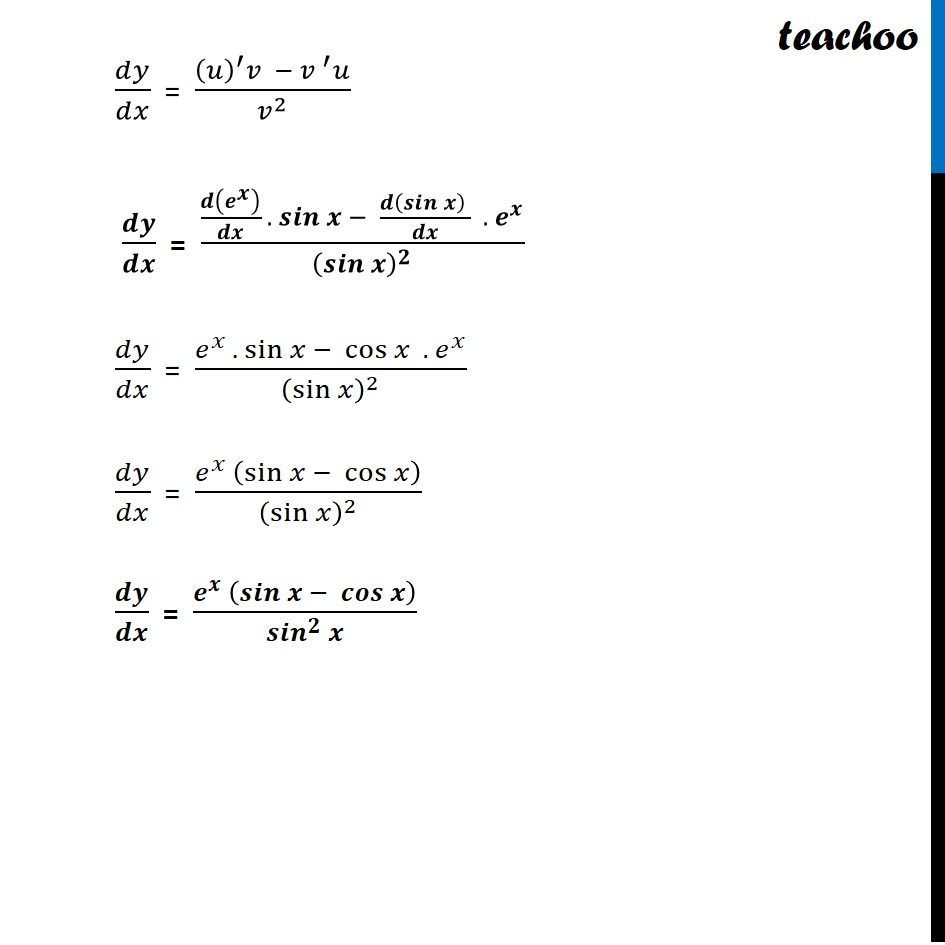Ex 5.4

Chapter 5 Class 12 Continuity and Differentiability
Serial order wiseLearn in your speed, with individual attention - Teachoo Maths 1-on-1 Class

### Transcript

Ex 5.4, 1 Differentiate 𝑤.𝑟.𝑡. 𝑥 in , 𝑒^𝑥/sin⁡𝑥 Let 𝒚 = 𝑒^𝑥/sin⁡𝑥 Let 𝑢 =𝑒^𝑥 & 𝑣 = sin⁡𝑥 ∴ 𝒚 = 𝒖/𝒗 Differentiating both sides 𝑤.𝑟.𝑡.𝑥 𝑑(𝑦)/𝑑𝑥 = 𝑑(𝑢/𝑣)/𝑑𝑥 𝑑𝑦/𝑑𝑥 = ((𝑢)^′ 𝑣 − 〖𝑣 〗^′ 𝑢)/𝑣^2 𝑑𝑦/𝑑𝑥 = ((𝑢)^′ 𝑣 − 〖𝑣 〗^′ 𝑢)/𝑣^2 𝒅𝒚/𝒅𝒙 = (𝒅(𝒆^𝒙 )/𝒅𝒙 . 𝒔𝒊𝒏⁡𝒙 − (𝒅(𝒔𝒊𝒏⁡𝒙 ) )/𝒅𝒙 . 𝒆^𝒙)/(𝒔𝒊𝒏⁡𝒙 )^𝟐 𝑑𝑦/𝑑𝑥 = (𝑒^𝑥 . sin⁡𝑥 − cos⁡𝑥 . 𝑒^𝑥)/(sin⁡𝑥 )^2 𝑑𝑦/𝑑𝑥 = (𝑒^𝑥 (sin⁡𝑥 − cos⁡𝑥 ))/(sin⁡𝑥 )^2 𝒅𝒚/𝒅𝒙 = (𝒆^𝒙 (𝒔𝒊𝒏⁡𝒙 − 𝒄𝒐𝒔⁡𝒙 ))/〖𝒔𝒊𝒏〗^𝟐⁡𝒙